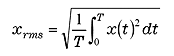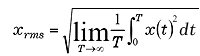FFT analyzer FAQWhat is Effective (RMS) value?Effective value is the amount of value expressing intensity of averaged time signal. It is found with the following equation (1) if the periodic signal x (t) is T.(1)

It is found with the following equation (2) if x (t) is non-periodic signal.(2)

Effective value is also called Rout Mean Square value from the above equations.

In a physical sense, if the power consumption of current-voltage and alternating-voltage is same as the power used when alternating-voltage is applied to a certain resistance R, the RMS of the current-voltage is E (V). RMS value of amplitude A sine wave(A sin ω t)is 0.707 A(0.707 = 1/√2).

Revised:2009/11/16